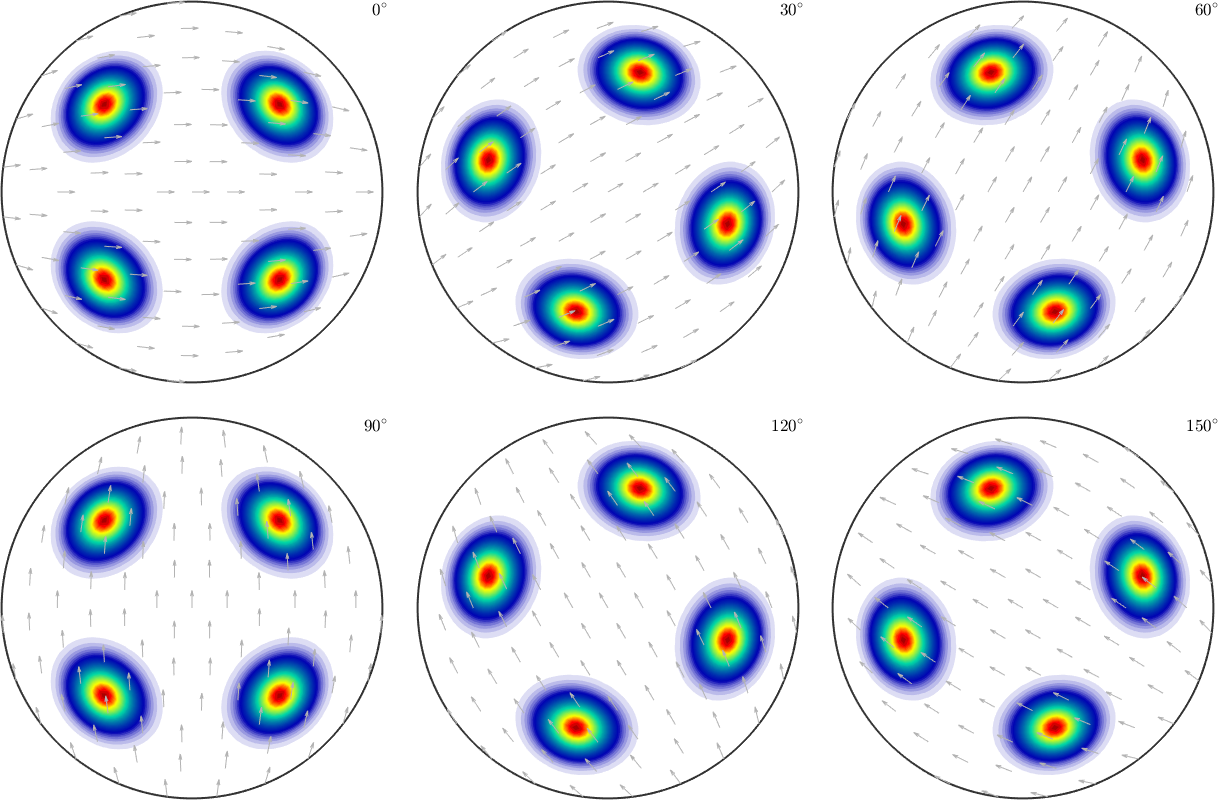ODF Tutorial edit page

## Theory

The orientation distribution function (ODF) is a function on the orientation space that associates to each orientation $$g$$ the volume percentage of crystals in a polycrystaline specimen that are in this specific orientation, i.e.,

$\mathrm{odf}(g) = \frac{1}{V} \frac{\mathrm{d}V(g)}{\mathrm{d}g}.$

In MTEX an entirely random texure will have an ODF constant to one. In other word the values of ODFs in MTEX can be interpreted as multiples of the random distribution (mrd).

## Computing an ODF from Individual Orientations

Individual orientations data may be obtained by experimental by EBSD, ACOM or 3d X-ray imaging; or from simulations, like VPSC. In the following we consider an EBSD map of an Titanium alloy.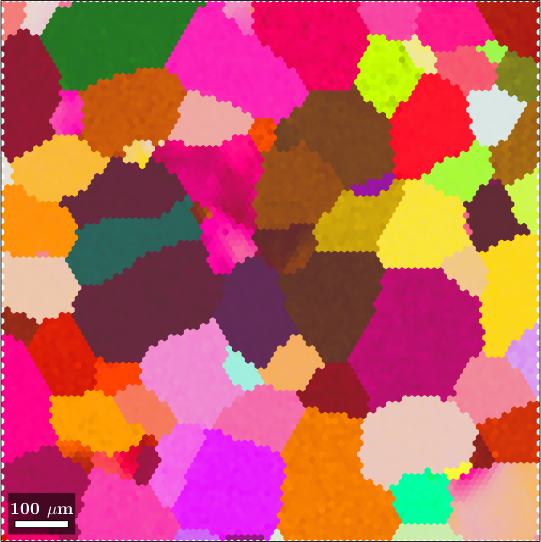Computing an ODF from individual orientations is done by kernel density estimation using the command calcDensity.

There many different ways to visualize ODF: Euler or sigma sections, thee dimensional plots, pole figures and inverse pole figures. The most common but not recommended way are sections with respect to the third Euler angle $$\varphi_2$$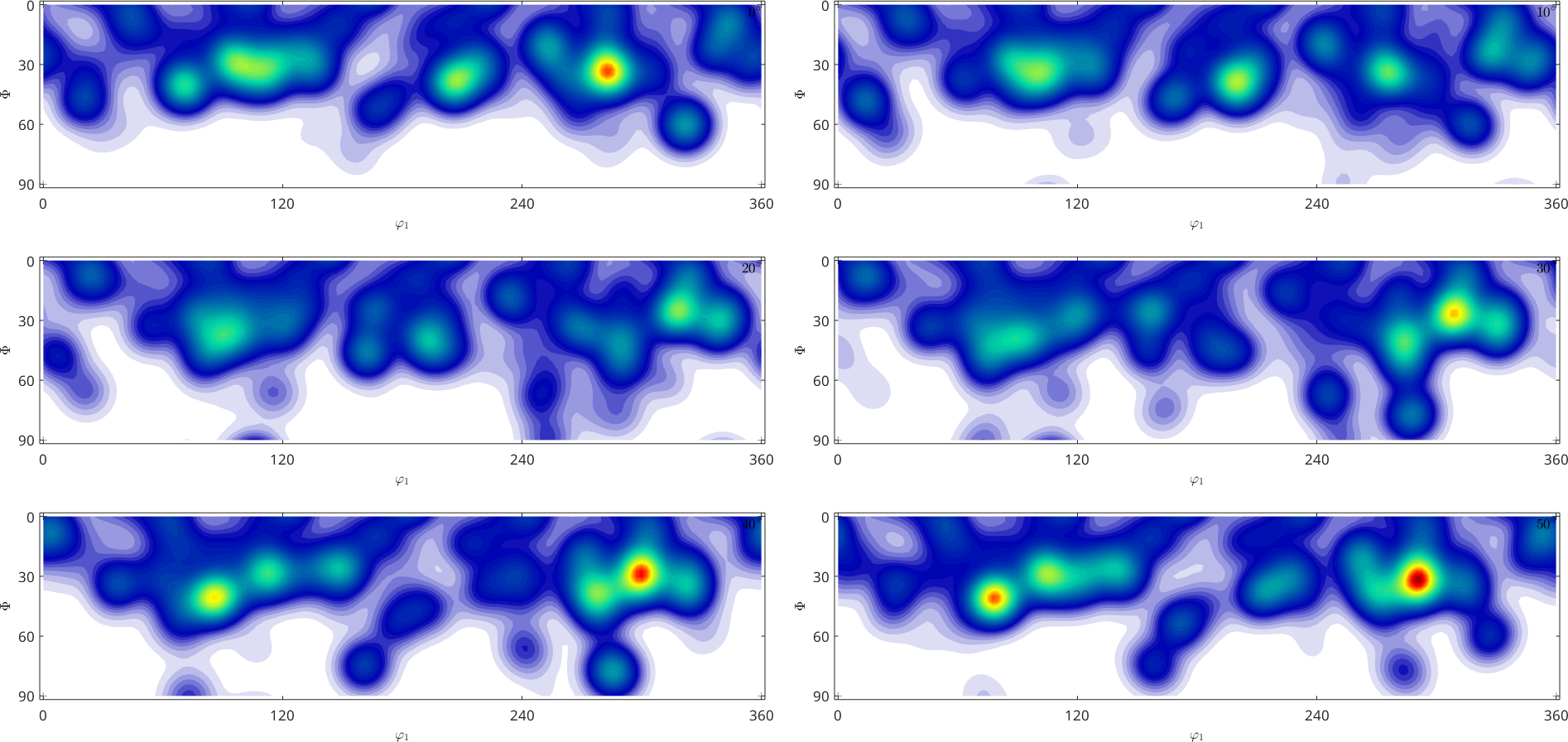## Computing an ODF from Pole Figure Data

Pole figure data arises when textured materials are measured via x-ray, neutron or syncrotron radiation. Generaly, for $$3$$ to $$10$$ diffraction planes specified by Miller indices $$(hk\ell)$$ diffraction intensities are measured at a spherical grid of specimen directions. In the example below each dot corresponds to one diffraction intensity at the plane indicated at the top of the spherical plots measured from the direction corresponding to the pixel position.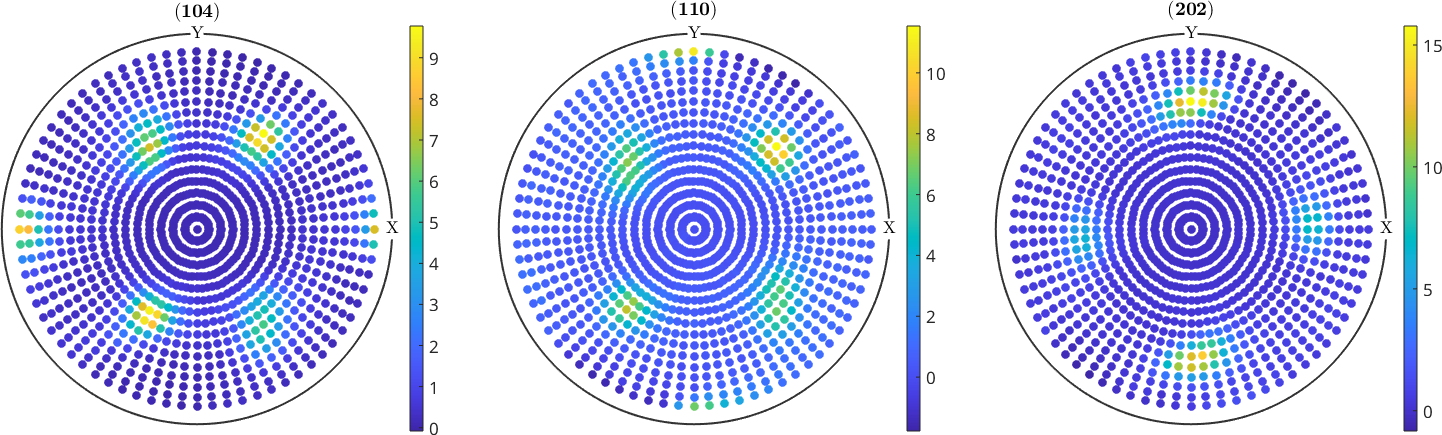The reconstuction of an ODF from pole figure data requires the solution of an ill posed inverse problem. This mean the reconstruction problem has in general not a unique solution, but there are several ODFs that correspond to the same set of pole figure data. MTEX applies some heuristics to identify among all solutions the physically most reasonable.

Once an ODF is reconstructed we can check how well its pole figures fit the measured pole figures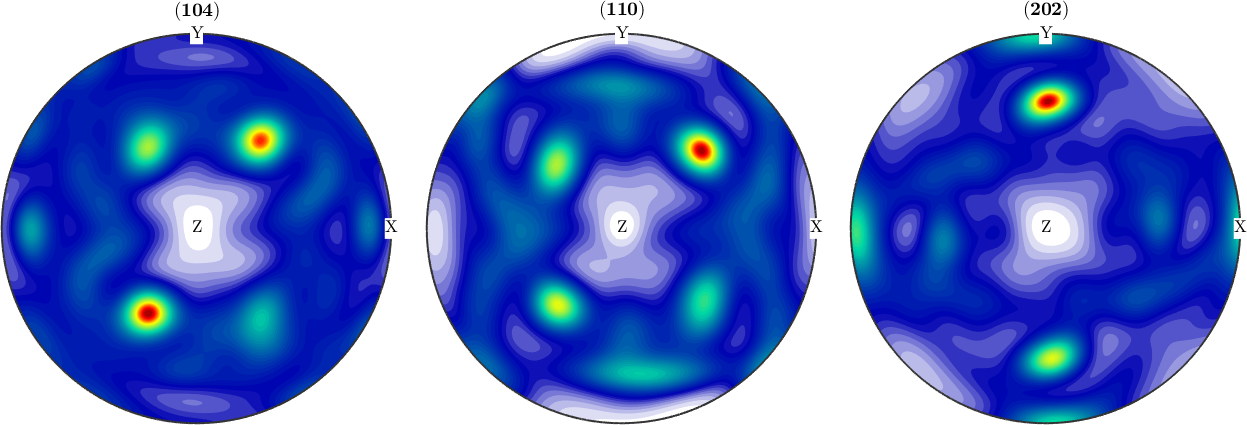## ODF Modelling

Besides from experimental data MTEX allows also the definition of model ODFS of different type. These include unimodal ODFs, fibre ODF, Bingham Distributed ODFs and any combination of such ODFs.